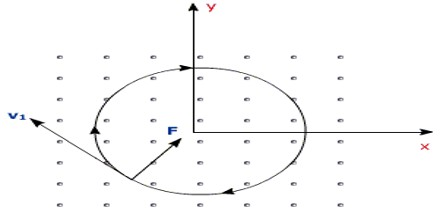Lorentz Force

Definition

Lorentz Force is a law of physics, particularly electromagnetism that describes the force interaction between magnetic fields of two charged particles. It was named after Hendrik Lorentz, a Dutch physicist during the 1800s who had great interest in the science of electromagnetism. A particle of charge q moving with velocity v in the presence of an electric field E and a magnetic field B experiences a force

F = qE + qv × B

Where, B is the strength of the magnetic field, E is the electric field,  q is the charge on the particle and  v is the velocity of the particle. It is expressed in “Newtons” as the unit of measure. According to this equation, if a particle of charge q moves with velocity v in the presence of an electric field E and a magnetic field B, then it will experience a reactive force. This reactive force is what is known as the Lorentz force.All charged particles encounter a force from an electric field, oriented in the direction of the field or the opposite direction, depending on the sign of the charge, while moving charged particles also encounter a force oriented at a right angle to both the direction of motion and the magnetic field. The Lorentz force is the driving force in electromagnets and is responsible for the Hall Effect.

The Lorentz Force equation was actually derived from the famous Maxwell’s equation. There are variations of this basic equation of the Lorentz force. Other equations, which could be found on other textbooks, describe the magnetic force on a current-carrying wire, electromotive force in a wire loop moving through a magnetic field, and the force on a charged particle which might be traveling near the speed of light.Significance of the Lorentz Force

While the modern Maxwell’s equations describe how electrically charged particles and currents or moving charged particles give rise to electric and magnetic fields, the Lorentz force law completes that picture by describing the force acting on a moving point charge q in the presence of electromagnetic fields. The Lorentz force law describes the effect of E and B upon a point charge. Charged particles are possibly coupled to other forces, notably gravity and nuclear forces. Thus, Maxwell’s equations do not stand separate from other physical laws, but are coupled to them via the charge and current densities. The response of a point charge to the Lorentz law is one aspect; the generation of E and B by currents and charges is another. The charged particles in a material medium not only respond to the E and B fields but also generate these fields. Complex transport equations must be solved to determine the time and spatial response of charges.

Equations of Lorentz Force

The force F acting on a particle of electric charge q with instantaneous velocity v, due to an external electric field E and magnetic field B is given by:

F = q(E+v×B)

A positively charged particle will be accelerated in the same linear orientation as the E field, but will curve perpendicularly to both the instantaneous velocity vector v and the B field according to the right-hand rule, in detail, if the fingers of the right hand are extended to point in the direction of v and are then curled to point in the direction of B, then the extended thumb will point in the direction of F.

The term qE is called the electric force, while the term qv × B is called the magnetic force. According to some definitions, the term “Lorentz force” refers specifically to the formula for the magnetic force, with the total electromagnetic force (including the electric force) given some other (nonstandard) name. This article will not follow this nomenclature: In what follows, the term “Lorentz force” will refer only to the expression for the total force.For a continuous charge distribution in motion, the Lorentz force equation becomes:

dF = dq(E +v×B)

Where, dF is the force on a small piece of the charge distribution with charge dq. If both sides of this equation are divided by the volume of this small piece of the charge distribution dV, the result is: f =ρ (E +v×B)

Where, f is the force density (force per unit volume) and ρ is the charge density (charge per unit volume).

Definition of E and B (Lorentz Force Law)

Lorentz force Law is used as the definition of the electric and magnetic fields E and B. To be specific, the Lorentz force is understood to be the following empirical statement:

The electromagnetic force F on a test charge at a given point and time is a certain function of its charge q and velocity v, which can be parameterized by exactly two vectors E and B, in the functional form: F =q (E +v×B)

As a definition of E and B, the Lorentz force is only a definition in principle because a real particle would generate its own finite E and B fields, which would alter the electromagnetic force that it experiences. In addition, if the charge experiences acceleration, as if forced into a curved trajectory by some external agency, it emits radiation that causes braking of its motion. See for example Bremsstrahlung and synchrotron light. These effects occur through both a direct effect and indirectly. Moreover, net force must include gravity, electroweak, and any other forces aside from electromagnetic force.